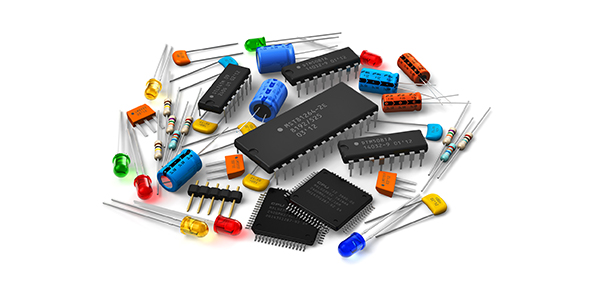Gibilisco - Inductance

20 Questions | Total Attempts: 924Settings• 1.
An inductor works by
• A.

Charging a piece of wire.

• B.

Storing energy as a magnetic field.

• C.

Choking off dc.

• D.

Introducing resistance into a circuit.

• 2.
Which of the following does not affect the inductance of an air-core coil, if all other factorsare held constant?
• A.

The frequency

• B.

The number of turns

• C.

The diameter of the coil

• D.

The length of the coil

• 3.
In a small inductance
• A.

Energy is stored and released slowly.

• B.

The current flow is always large.

• C.

The current flow is always small.

• D.

Energy is stored and released quickly.

• 4.
A ferromagnetic core is placed in an inductor mainly to
• A.

Increase the current carrying capacity.

• B.

Increase the inductance.

• C.

Limit the current.

• D.

Reduce the inductance.

• 5.
Inductors in series, assuming there is no mutual inductance, combine
• A.

Like resistors in parallel.

• B.

Like resistors in series.

• C.

Like batteries in series with opposite polarities.

• D.

In a way unlike any other type of component.

• 6.
Suppose two inductors are connected in series, without mutual inductance. Their values are33 mH and 55 mH. What is the net inductance of the combination?
• A.

1.8 H

• B.

22 mH

• C.

88 mH

• D.

21 mH

• 7.
If the same two inductors (33 mH and 55 mH) are connected in parallel without mutualinductance, the combination will have a value of
• A.

1.8 H.

• B.

22 mH.

• C.

88 mH.

• D.

21 mH.

• 8.
Suppose three inductors are connected in series without mutual inductance. Their values are4.00 nH, 140 µH, and 5.07 H. For practical purposes, the net inductance will be very close to
• A.

4.00 nH.

• B.

140 µH.

• C.

5.07 H.

• D.

None of the above.

• 9.
Suppose the three inductors mentioned above are connected in parallel without mutualinductance. The net inductance will be close to
• A.

4.00 nH.

• B.

140 µH.

• C.

5.07 H.

• D.

None of the above.

• 10.
Suppose two inductors, each of 100 µH, are connected in series, and the coefficient ofcoupling is 0.40. The net inductance, if the coil fields reinforce each other, is
• A.

50.0 µH.

• B.

120 µH.

• C.

200 µH.

• D.

280 µH.

• 11.
If the coil fields oppose in the foregoing series-connected arrangement, assuming thecoefficient of coupling does not change, the net inductance is
• A.

50.0 µH.

• B.

120 µH.

• C.

200 µH.

• D.

280 µH.

• 12.
Suppose two inductors, having values of 44.0 mH and 88.0 mH, are connected in series witha coefficient of coupling equal to 1.0 (the maximum possible mutual inductance). If their fieldsreinforce, the net inductance is approximately
• A.

7.55 mH.

• B.

132 mH.

• C.

194 mH.

• D.

256 mH.

• 13.
If the fields in the previous situation oppose, assuming the coefficient of coupling does notchange, the net inductance will be approximately
• A.

7.55 mH.

• B.

132 mH.

• C.

194 mH.

• D.

256 mH.

• 14.
With permeability tuning, moving the core further into a solenoidal coil
• A.

Increases the inductance.

• B.

Reduces the inductance.

• C.

Has no effect on the inductance, but increases the current-carrying capacity of the coil.

• D.

Raises the frequency

• 15.
A significant advantage, in some situations, of a toroidal coil over a solenoid is the fact that
• A.

The toroid is easier to wind.

• B.

The solenoid cannot carry as much current.

• C.

The toroid is easier to tune.

• D.

The magnetic flux in a toroid is practically all within the core.

• 16.
A major feature of a pot core inductor is
• A.

High current capacity.

• B.

Large inductance in small volume.

• C.

Excellent efficiency at very high frequencies.

• D.

• 17.
As an inductor core material, air
• A.

Has excellent efficiency.

• B.

Has high permeability.

• C.

Allows large inductance to exist in a small volume.

• D.

Has permeability that can vary over a wide range.

• 18.
At a frequency of 400 Hz, which is in the AF range, the most likely form for an inductorwould be
• A.

Air-core.

• B.

Solenoidal.

• C.

Toroidal.

• D.

Transmission-line.

• 19.
At a frequency of 95.7 MHz, which is in the frequency-modulation (FM) broadcast band andis considered part of the very high frequency (VHF) radio spectrum, a good form for an inductorwould be(a) (b) (c) (d)
• A.

Air-core.

• B.

Pot core.

• C.

Either air-core or pot core.

• D.

Neither air-core nor pot core.

• 20.
A transmission-line inductor made from coaxial cable having velocity factor of 0.66 andworking at 450 MHz, which is in the ultrahigh frequency (UHF) radio spectrum, should, in orderto measure less than 1⁄4 electrical wavelength, be cut shorter than
• A.

16.7 m.

• B.

11 m.

• C.

16.7 cm.

• D.

11 cm.

Related TopicsBack to top